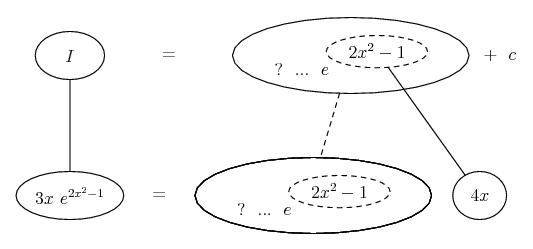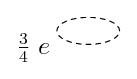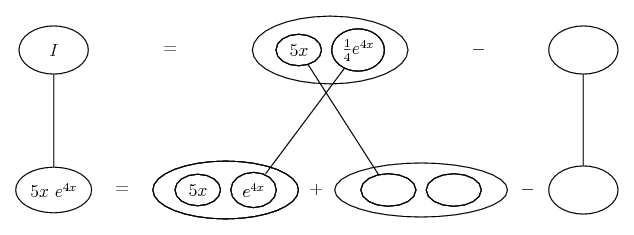# Thread: 'Integrate with respect to x'

1. ## 'Integrate with respect to x'

Hi
I am helping out a friend at my dad's work with his maths (its part of his apprenticeship course). I've just finished doing A-Level maths myself, over a 12 month period.

The question i have is actually in the attachment. Parts 3 a) and b)

With this, i know that dy/dy of e^x is e^x, but not sure how to begin tackling this question, and is possibly above my level i'm not sure. Its for an engineering course so i don't think it was on my syllabus. Any beginning help for either question would be great

Thanks

2. You need to use integration by parts to find the answer to these problems.

It is $\displaystyle \int uv' = uv - \int u'v$

3. 3 (a) ... simple substitution. let $\displaystyle u = 2x^2 - 1$

3 (b) ... integration by parts

4. As Mr. Pickles said use integration by parts. I would use

$\displaystyle u=e^{2x^2-1}$
$\displaystyle u'=4xe^{2x^2-1}$
$\displaystyle v=\frac{3x^{2}}{2}$
$\displaystyle v'=3x$

5. I like being called Mr Pickles, its funny. Can a mod change that for me (instead of 'Super Member') please??

6.Originally Posted by pickslidesI like being called Mr Pickles, its funny. Can a mod change that for me (instead of 'Super Member') please??
Gee I suck today. I totally thought that said pickles. Wow. . .this is why I stink at math, my reading skills are atrocious.

7. I agree that reading skills can affect your ability to analyse problems mathematically.

Others on this forum have called me Mr Pickles so don't feel bad.

8. Hi again
What is the best way to tackle part a)? Is it by substitution or by integration by parts?

With the substitution option for a), it would lead to...3xe^u, do i then use the 'differentiation of a product rule' ?

I have a page in my textbook regarding integration by parts but cannot make sense of the example and relate it to part b). I've attached the example, or basically its the first half of the page. I've read it over and over but can't seem to 'get it'. It doesn't help either with the 'limits'.

I hope i'm on the right track with a) but not too sure about b)

9. $\displaystyle \int_0^1 3x e^{2x^2-1} \, dx$

$\displaystyle u = 2x^2 - 1$

$\displaystyle du = 4x \, dx$

$\displaystyle \frac{3}{4} \int_0^1 4x e^{2x^2-1} \, dx$

substitute and reset the limits of integration ...

$\displaystyle \frac{3}{4} \int_{-1}^1 e^u \, du$

finish.

---------------------------------------------------------

$\displaystyle \int 5xe^{4x} \, dx$

$\displaystyle u = 5x$

$\displaystyle dv = e^{4x} \, dx$

$\displaystyle du = 5 \, dx$

$\displaystyle v = \frac{e^{4x}}{4}$

$\displaystyle uv - \int v \, du$

$\displaystyle \frac{5x e^{4x}}{4} - \int \frac{5e^{4x}}{4} \, dx$

$\displaystyle \left[\frac{5x e^{4x}}{4} - \frac{5e^{4x}}{16}\right]_0^1$

evaluate.

10. Hi thanks for the post
A couple of things i didn't understand on part a)...where does the 3/4 come from? And also, i've jsut flicked thru my notes, why do the limits reset?

On b), do i think just insert values of x?

11.Originally Posted by JontyPHi thanks for the post
A couple of things i didn't understand on part a)...where does the 3/4 come from?

$\displaystyle u = 2x^2 - 1$

$\displaystyle du = 4x \, dx$

But there is no "4x" term in

$\displaystyle \int_0^1 3x e^{2x^2-1} \, dx$

So Skeeter has cleverly taken out a constant of $\displaystyle \frac{3}{4}$ so "du" can be used in the problem. Now the substitution can be made.

$\displaystyle \frac{3}{4} \int_0^1 4x e^{2x^2-1} \, dx$Originally Posted by JontyPwhy do the limits reset?
Because the variable has changed from x to u. The relationship between these variables is used to find the new limits.

12.Originally Posted by JontyPOn b), do i think just insert values of x?
Yep like this

$\displaystyle \left[\frac{5(1) e^{4(1)}}{4} - \frac{5e^{4(1)}}{16}\right] - \left[\frac{5(0) e^{4(0)}}{4} - \frac{5e^{4(0)}}{16}\right] = \dots$

13. Just in case a picture helps...
Substitution is for working backwards through the chain rule for differentiation...If the dashed balloon is the inner function of a composite function, then the rule tells us to differentiate the top balloon with respect to the dashed balloon inside it, and then multiply by the derivative of the dashed balloon. So the straight continuous line is differentiation (downwards) with respect to x, and the straight dashed line with respect to the dashed balloon expression.
So 3x e to the power 2x - 1 could be the result of differentiation by the chain rule...We just need to multiply by a number that will make the lower equals sign valid... (that mystery fraction!).
And so then integratewith respect to the dashed balloon.

The challenge of integration by parts is to work backwards through the product rule for differentiation...... we want to fill out this product-rule shape usefully, but starting in one of the bottom corners instead of - as happens in differentiation, the top one. Once you've started in the right way, as here...... it's really a matter of following through. Fill in the rest of the product-rule shape, then you'll need cancel the addition you just entered, to make the lower equals sign valid, again.
_______________________________

Don't integrate - balloontegrate!

Balloon Calculus Forum

integrate, respect